# Problem 57983. Easy Sequences 109: Summation of Derivatives of a Trigonometric Function

A trigonometric function,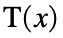, is defined as follows:where: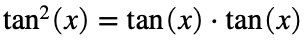; and
In this problem we are asked to evaluate the following summation:where: x is a real number;
n is an integer;or the k-th derivative of T with respect to x; and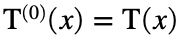.
For example for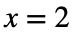and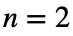, we have:
>> syms x;
>> T = (1+2*tan(x)-tan(x)^2) / (1+tan(x)^2);
>> S = T/2^0 + diff(T)/2^1 + diff(T,2)/2^2;
>> s = vpa(subs(S,x,2))
s = 0.10315887444431633673347091141408;
Please present the final output rounded-off to 6 decimal places. Therefore the final answer is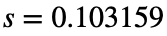.
-------------------------
NOTE: Symbolic toolbox is not available to Cody players. It is possible to do numerical differentiation in Matlab without symbolic toolbox. Just make sure that the output would be accurate within 6 decimal places of the 'exact' value obtained using syms, as shown above.

### Solution Stats

62.5% Correct | 37.5% Incorrect
Last Solution submitted on Jun 05, 2023

### Community Treasure Hunt

Find the treasures in MATLAB Central and discover how the community can help you!

Start Hunting!